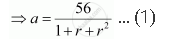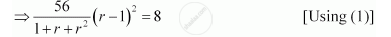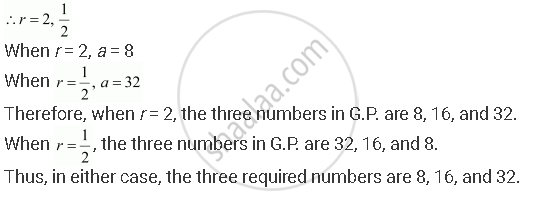# The Sum of Three Numbers in G.P. is 56. If We Subtract 1, 7, 21 from These Numbers in that Order, We Obtain an Arithmetic Progression. Find the Numbers. - Mathematics

The sum of three numbers in G.P. is 56. If we subtract 1, 7, 21 from these numbers in that order, we obtain an arithmetic progression. Find the numbers.

#### Solution

Let the three numbers in G.P. be aar, and ar2.

From the given condition, a + ar + ar2 = 56

⇒ a (1 + r + r2) = 56a – 1, ar – 7, ar2 – 21 forms an A.P.

∴(ar – 7) – (a – 1) = (ar2 – 21) – (ar – 7)

⇒ ar – a – 6 = ar– ar – 14

ar– 2ar + a = 8

ar– ar – ar + a = 8

a(r+ 1 – 2r) = 8

⇒ (r – 1)2 = 8 … (2)⇒7(r2 – 2r + 1) = 1 + r + r2

⇒7r2 – 14 r + 7 – 1 – r – r2 = 0

⇒ 6r2 – 15r + 6 = 0

⇒ 6r2 – 12r – 3r + 6 = 0

⇒ 6r (r – 2) – 3 (r – 2) = 0

⇒ (6r – 3) (r – 2) = 0Concept: Relationship Between A.M. and G.M.
Is there an error in this question or solution?

#### APPEARS IN

NCERT Class 11 Mathematics
Chapter 9 Sequences and Series
Q 10 | Page 199